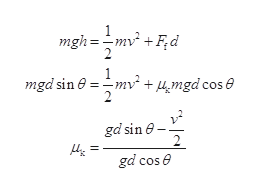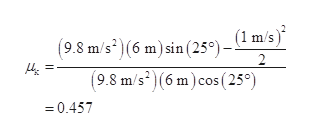# A 10kg box slides down a 6 m long ramp that makes an angle of 25 degrees with the ground. Atthe bottom of the ramp, the box is moving 1m/s. If the specific heat is 2 joules per degree kg,How much did the temperature of the box change by and what is the coefficient of friction?

Question
11 views

A 10kg box slides down a 6 m long ramp that makes an angle of 25 degrees with the ground. At
the bottom of the ramp, the box is moving 1m/s. If the specific heat is 2 joules per degree kg,
How much did the temperature of the box change by and what is the coefficient of friction?

check_circle

Step 1

Write the expression for conservation of energy for the given systemhelp_outlineImage Transcriptionclosemgh=-mv +Fd 1 =mv + mgdcos e 2 mgd sin e gd sin 2 gd cos fullscreen
Step 2

Substitute the valueshelp_outlineImage Transcriptionclose(1 m/s) (9.8 m/s2)(6 m) sin (25°)- 2 (9.8 m/s2(6 m) cos (250) =0.457 fullscreen
Step 3

Work done by friction is converted into heat energ...

### Want to see the full answer?

See Solution

#### Want to see this answer and more?

Solutions are written by subject experts who are available 24/7. Questions are typically answered within 1 hour.*

See Solution
*Response times may vary by subject and question.
Tagged in